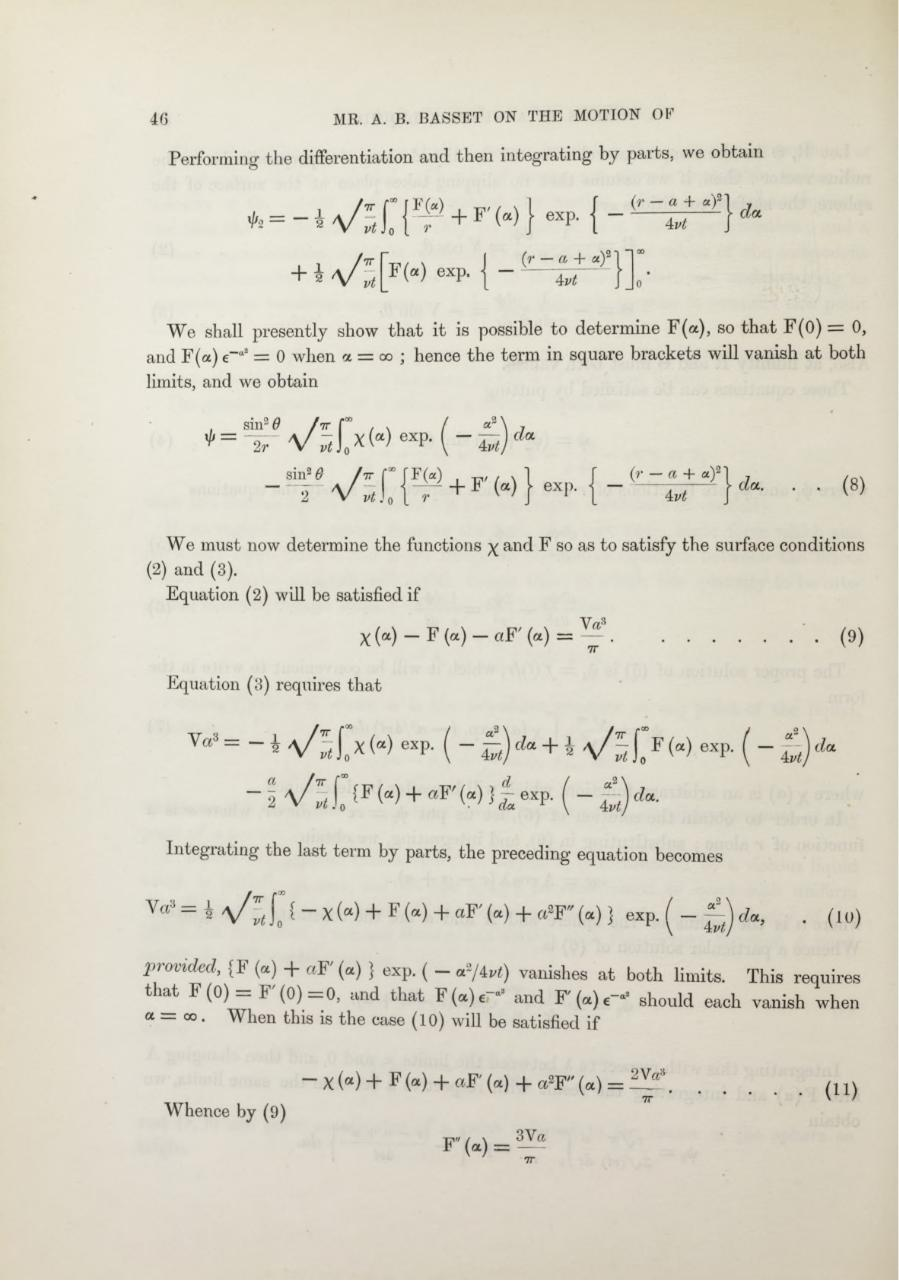# PDF Archive

Easily share your PDF documents with your contacts, on the Web and Social Networks.

## A. B. Basset. On the motion of a sphere in a viscous liquid.pdfPage 1 2 3 45621

#### Text preview

MR. A. B. BASSET ON THE MOTION OF

Performing the differentiation and then integrating by paits, we obtain

* = - *

a/ s

+ i

C { ^ +

f'w

F (“ ) exP- { ~

(r —
a+ a)2
4vt
} exp- { “
(r — a + a)2
4

We shall presently show th at it is possible to determine F(a), so th a t F(0) = 0,
and F(a) e_“2= 0 when a = oo ; hence the term in square brackets will vanish at both
limits, and we obtain
XIJ =

sin2 6

\ / ^ l 0x ( “ ) ex p - { - £ ) d a

± «yi
4vt

( 8)

We must now determine the functions
(2) and (3).
Equation (2) will be satisfied if

x and F

X( « ) - F ( a ) - « F &gt; ) = ^

........................................... (9)

Equation (3) requires that

= - i

x w exp- ( - £

) + * \ / } t \ y («) «xp - ( - Q

“ I V s 10 1F w + aW (?)} £ exp- ( - £ ) * * ■
Integrating the last term by parts, the preceding equation becomes
|

\ / vt| 0 { “ X (a ) + F (a) + aF ' (a) -f a 2F&quot; ( a ) } exp. ^ —

.

(10)

provided, {F (a) + aF ' (a) } exp. ( - «»/4«*) vanishes at both limits. This requires
that 4 (0) _ F'(0) = 0 , and that Y(a,)e~'* and F' (a) e-a2should each vanish when
a — oo. When this is the case (10) will be satisfied if
- X (a ) + F (a) + aF' (a) + a 2F&quot; (a) = ^
Whence by (9)

7T

.

(in